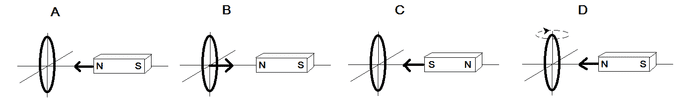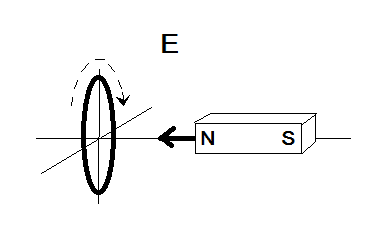# SAT II Physics -- Induced current in loop (Faraday's law)

## Homework Statement

A loop of wire and a bar magnet are moving relative to one another. Which motion in the diagrams shown below will NOT induce a current in the loop?## Homework Equations

Lenz's Law: A changing magnetic field can induce a current in a direction that produces its own magnetic field that opposes the change.

Faraday's Law: A mathematical expression of Lenz's law that states the induced voltage is minus the rate of change of magnetic flux with respect to time.

## The Attempt at a Solution

[/B]
It is very clear that A, B, and C all have a changing magnetic flux since the field gets stronger as the magnet gets closer to the loop.

In D the angle of loop is changing, and since flux only counts the number of magnetic lines perpendicularly cutting the plane, as the loop rotates the flux changes.

So the answer is E by process of elimination. Yet in E doesn't the flux change too, since the magnet is getting closer? How does spinning the loop affect the change in flux?

I researched a bit and think it might have something to do with faraday's paradox but I did not understand it.

## Answers and Replies

Simon Bridge
Science Advisor
Homework Helper
The current is the motion of charges along a wire ... but what is the wire is moving at the same speed as the charges? Is there still a current?
Draw an ammeter in the loop and see if that helps... the current is the amount of charge passing through the ammeter per unit time.

The current is the motion of charges along a wire ... but what is the wire is moving at the same speed as the charges? Is there still a current?
Draw an ammeter in the loop and see if that helps... the current is the amount of charge passing through the ammeter per unit time.

I assume you mean if the wire rotates in a direction opposite to the motion of charges?

Also, wouldn't it depend on the reference frame? In the frame of the wire there would be a current but in other frames there wouldn't be?

Further, doesn't this violate faraday's law since the magnetic flux is changing yet no current is produced.

Simon Bridge
Science Advisor
Homework Helper
Actually - I think there is an error in E. I think the arrow on the magnet shouldn't be there.
Unless you have covered some material on frame dependence of current?

The current is really a bunch of negative charge moving wrt the wire, which is positively charged (the net being neutral) ... in this case, the negative charge is being induced to rotate about the center of the loop. If the loop is rotated in pace with the negative charges, then... (stay in the reference frame of the center of the loop if you like...)

So to check - in diagram E: is the loop being spun in the direction of the induced conventional current or opposite?

Actually - I think there is an error in E. I think the arrow on the magnet shouldn't be there.
Unless you have covered some material on frame dependence of current?

The current is really a bunch of negative charge moving wrt the wire, which is positively charged (the net being neutral) ... in this case, the negative charge is being induced to rotate about the center of the loop. If the loop is rotated in pace with the negative charges, then... (stay in the reference frame of the center of the loop if you like...)

So to check - in diagram E: is the loop being spun in the direction of the induced conventional current or opposite?

By Faraday's law, without any rotation the induced current would be counter clockwise.
Hence it appears that the clockwise rotation of the wire is opposite to what the direction of the induced current would be.

I still don't get how if no current were there this isn't a violation of Farday's law though.

rude man
Homework Helper
Gold Member
If the arrow indicates magnet motion then I agree, there will still be induced current. The circular motion of the loop does not affect current production.

But if that arrow is there by mistake, the story is different.

If the arrow indicates magnet motion then I agree, there will still be induced current. The circular motion of the loop does not affect current production.

But if that arrow is there by mistake, the story is different.

How would the story change if it were indeed a mistake (as I am inclined to believe, considering the relatively low quality of many SAT prep books). I assume it might relate the homopolar generator?

rude man
Homework Helper
Gold Member
How would the story change if it were indeed a mistake (as I am inclined to believe, considering the relatively low quality of many SAT prep books). I assume it might relate the homopolar generator?
Never heard of a homopolar generator.

BUT - if the bar magnet were stationary then just apply faraday's law to the loop! Does the motion of the loop really affect the law's consequences?

Simon Bridge
Science Advisor
Homework Helper
I the loop rotates in the opposite direction of the induced conventional current.

IMO it would be a violation of Faraday... rude man is correct to test this by seeing what happens if you remove the motion of the magnet.

Like I say, I'm inclined to think this is a mistake: the author probably forgot to remove the arrow when copying and pasting the magnet pic.